##### Managerial Economics For Dummies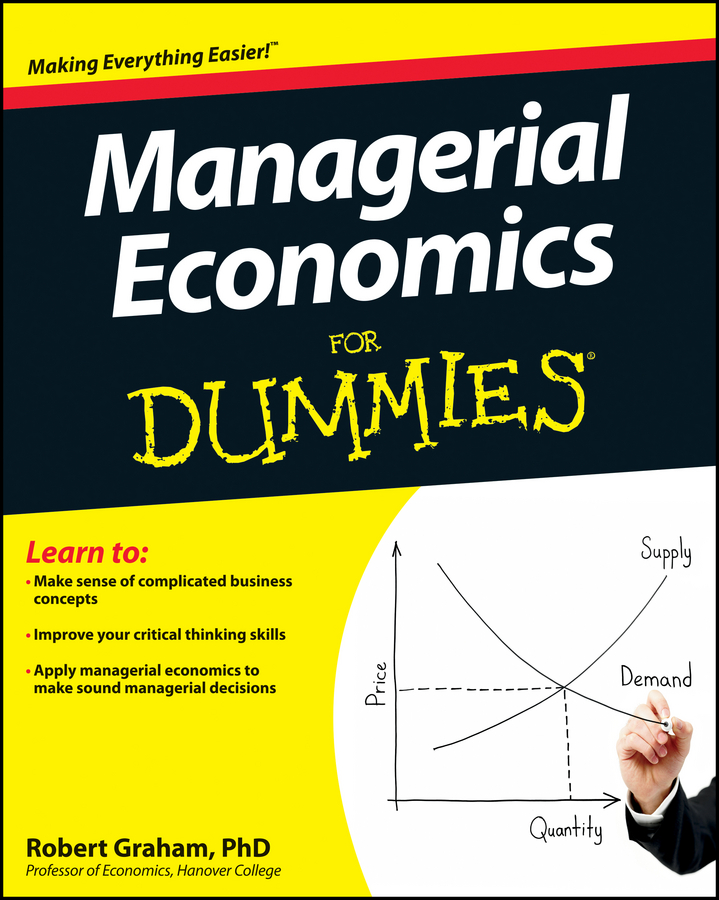Managerial economics allows firms to compute the price of a product that would maximize profits. To do this, they need total revenue and total cost. Total revenue equals price multiplied by the quantity sold, or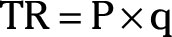In this equation, P represents the commodity’s price as determined by supply and demand in the market. For a perfectly competitive market, this price is a constant — it doesn’t change regardless of the quantity of output produced by your firm. You must determine the quantity of output, q0, that maximizes your firm’s profit given the market price P.

Total revenue is illustrated as an upward-sloping straight line. Because your firm is a price taker in perfect competition, the slope of the total revenue function is a constant and corresponds to the market-determined price.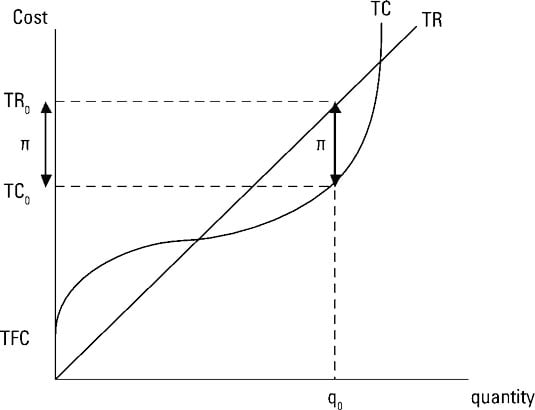Total cost has two components — total fixed cost and total variable cost. Total fixed cost is a constant, so even if your firm shuts down and produces zero units of output, it still incurs total fixed cost. In the illustration, total fixed cost corresponds to the point where the total cost curve intersects the vertical axis at TFC.

As the quantity of output produced increases, total cost increases at a decreasing rate. This fact indicates the total cost curve is becoming flatter due to diminishing returns. Inevitably, however, total cost begins increasing at an increasing rate; or, in other words, the total cost curve becomes steeper, as illustrated.

Total profit equals total revenue minus total cost, or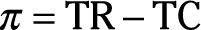Total profit is maximized at the output level where the difference between total revenue and total cost is greatest. In the illustration, this occurs at the output level q0. At the output level q0, total revenue equals TR0, total cost equals TC0, and total profit is the difference between them.

On the graph, total profit, ð, is the vertical distance between TR0 and TC0, and this vertical distance is at its greatest at q0.

Economists use the terms profit and economic profit interchangeably. Economic profit is defined as the difference between total revenue and the explicit plus implicit costs of production. As an equation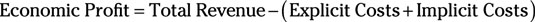The explicit costs plus implicit costs include every cost associated with production, including the opportunity cost of your time and financial investment. Therefore, if economic profit equals zero, you stay in business. Zero economic profit means you’re receiving exactly as much income in this situation as you will in your next best alternative.

Zero economic profit is okay. Positive economic profit is even better. Negative economic profit is always bad.